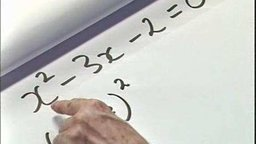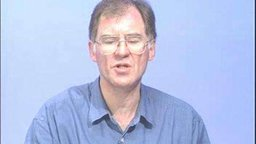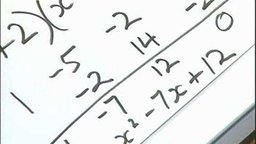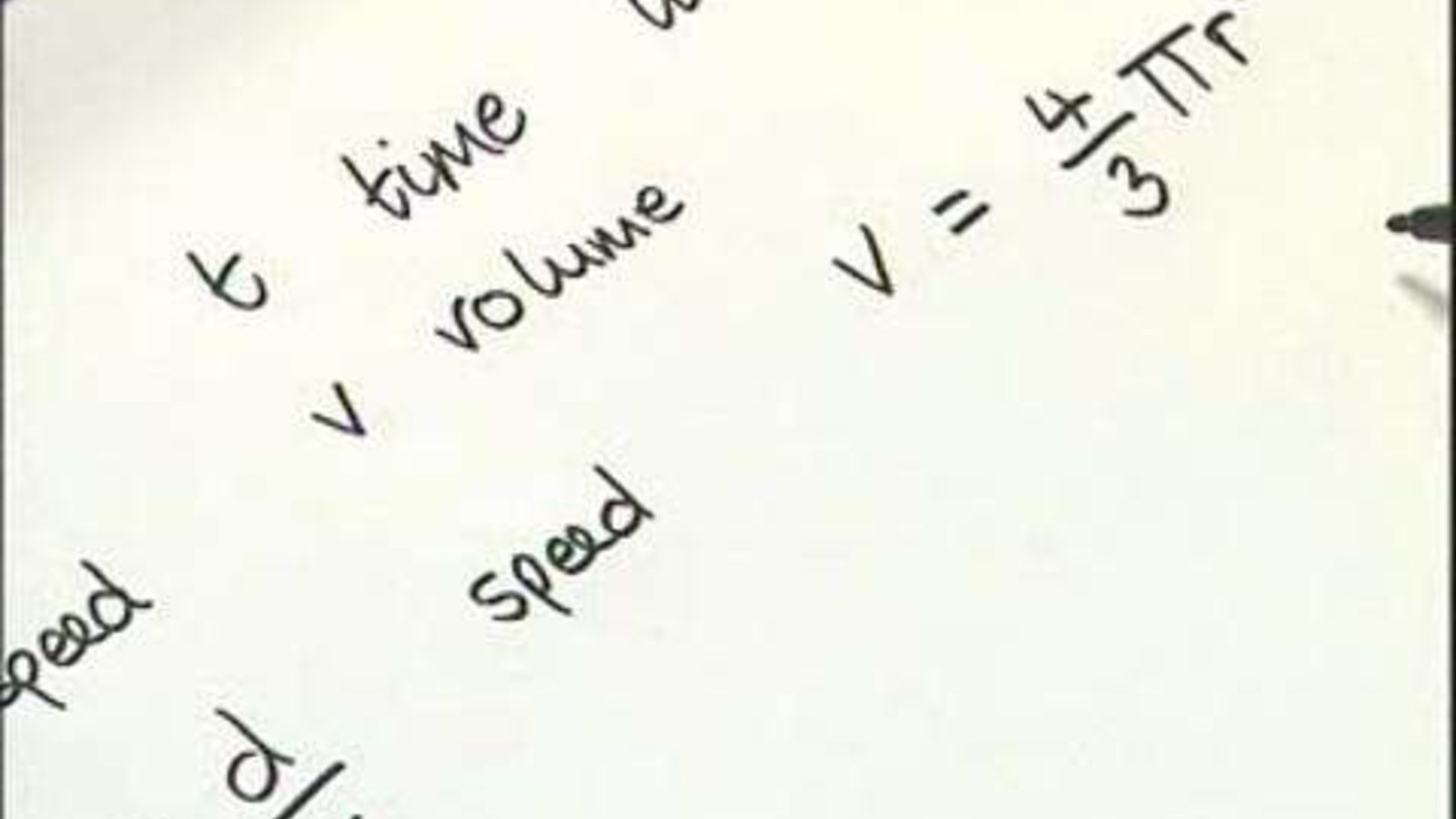# Algebra

2007The 18 videos in this collection focus on all aspects of Algebra, from logarithms to quadratic equations and partial fractions.

Supplier
FENC

### In This Series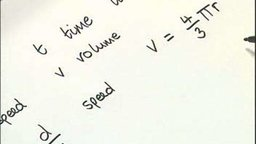Mathematical LanguagePowers or Indices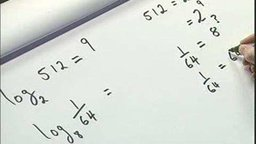Logarithms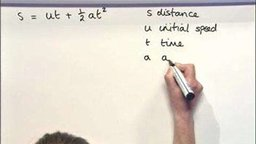Substitution and Formulae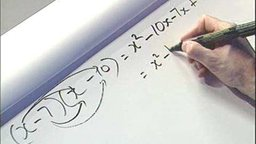Expanding and Removing Brackets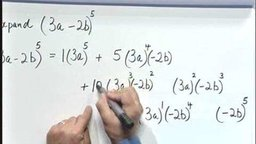Pascal's Triangle and the Binomial Theorem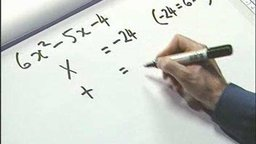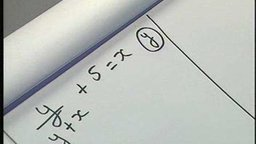Transposition of FormulaeLinear Equations in One VariableCompleting the Square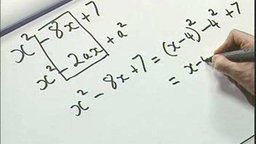Completing the Square (max, min)